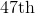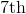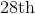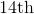# GED Math : Representing Data

## Example Questions

### Example Question #21 : Representing Data

The following graph shows the distribution of students at a high school that has a student population of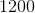: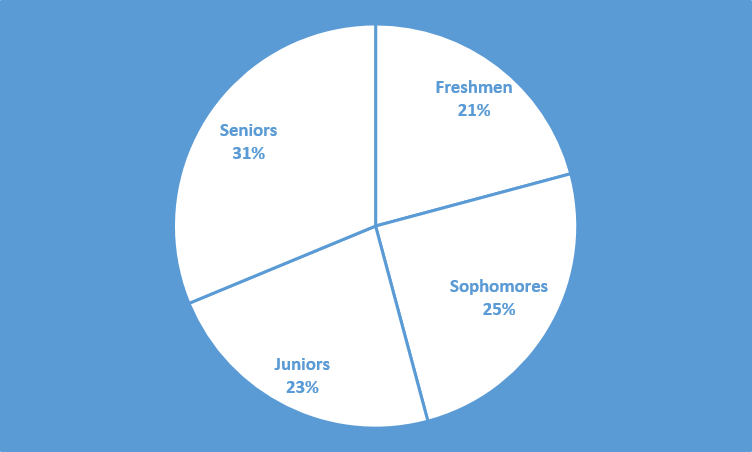How many freshmen does this school have?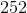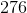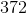Explanation:From the chart, we can see that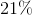of the school population is freshmen. Thus, we will need to findof.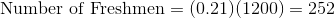### Example Question #22 : Representing Data

The following graph shows the distribution of students in a high school that has a student population of:In degrees, what is the central angle of the sector labeled "Juniors"? Round to the nearest tenths place.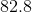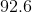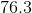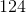Explanation:The sector labeled "Juniors" takes up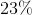of the circle. Thus, its central angle must beof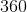, the number of total angles in a circle.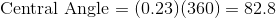The central angle must bedegrees.

### Example Question #23 : Representing Data

The following graph shows the distribution of students in a high school that has a student population of:How many more juniors and seniors are there than freshmen and sophomores?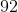Explanation:The juniors and seniors make up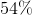of the school's population. Thus, we can find the number of juniors and seniors.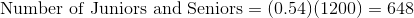The freshmen and sophomores make up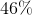of the school's population. Thus, we can find the number of freshmen and sophomores.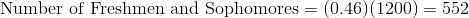Subtract these two numbers to find how many more juniors and seniors there are than freshmen and sophomores.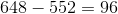There aremore juniors and seniors than there are freshmen and sophomores.

### Example Question #1 : Bar Graphs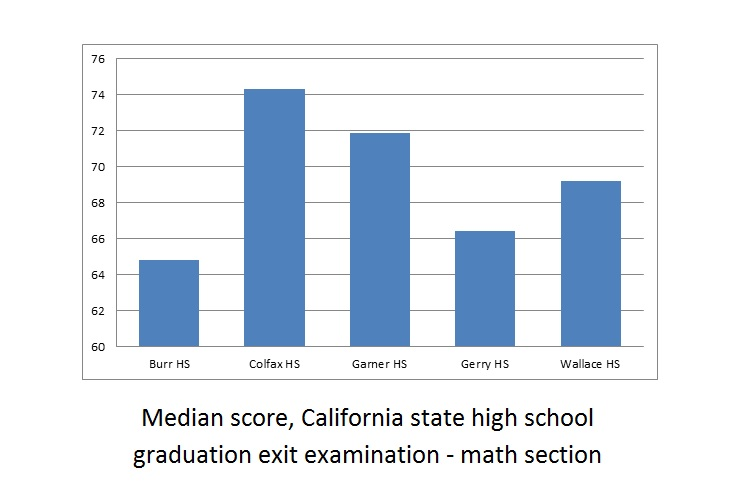A school district includes five high schools - Burr, Colfax, Garner, Gerry, and Wallace. Seniors at all five high schools took the California state high school exit examination this year - the results are reflected in the above bar graph.

Which of the following questions cannot be answered about the math scores of the five schools by examining the above graph?

Of the five schools, which school's median score improved the most from last year?

Of the five schools, how many had median math scores above 70 this year?

What was the difference this year between the median math score of the highest-performing school of the five and that of the lowest-performing school?

Of the five schools, which school's median math score was the lowest this year?

Of the five schools, which school's median score improved the most from last year?

Explanation:

The question "Of the five schools, which school's median score improved the most from last year?" requires knowledge of last year's median math scores, which are not given by the graph. The other three questions only require knowledge of this year's scores, which are given.

### Example Question #2 : Bar GraphsRefer to the above bar graph. The exit examination was given to all high school seniors in the above five schools.

Juanita attended Wallace High School and scored a 75 on the math portion. Wallace High School had 188 seniors take the examination. How many seniors could Juanitia have conceivably outscored?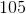Explanation:

The median score at Wallace was 69, so Juanita scored above the median. By definition, she outscored at least half the seniors, which means that she must have outscored at least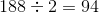of them. The correct response must be greater than or equal to 94, and the only choice that fits that criterion is 105.

### Example Question #3 : Bar GraphsRefer to the above bar graph.

Three cousins took this examination this year- Carlos scored 67, Alberto scored 70, and Julio scored 71. All three attended the same high school and all three scored below the median for their school. Which of the following high schools could they have attended?

Garner

Burr

Wallace

Gerry

Garner

Explanation:

Julio scored the highest of the three with 71, so we are looking for a high school whose median score was above 71. Of the four choices, only Garner fits this criterion.

### Example Question #4 : Bar Graphs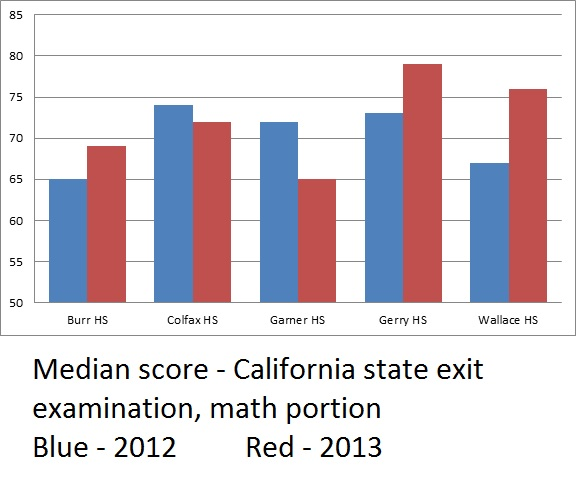The above bar graph compares the median math scores from both 2012 and 2013 for an examination administered in the five high schools of a school district.

How many of the five schools did not see their median math score reach at least 70 in either 2012 or 2013?

Three

One

None

Two

One

Explanation:

Of the five schools, only Burr had median scores below 70 in both 2012 and 2013. The correct choice is "one".

### Example Question #5 : Bar Graphs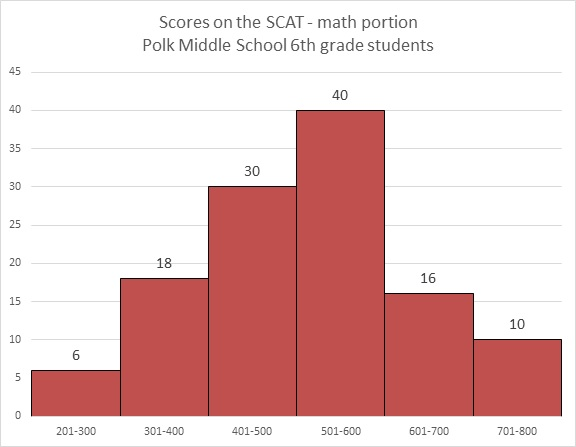Refer to the above bar graph.

How many students at Polk Middle School scored higher than 600 on the math portion of the SCAT?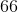Explanation:

The number of students who scored in the 601-700 range is 16; in the 701-800 range, 10. Add them to get 26 students total.

### Example Question #6 : Bar GraphsRefer to the above bar graph.

What percent of the students achieved a score above 500?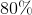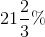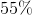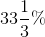Explanation:

40 students achieved a score of 501-600; 16 achieved a score of 601-700; 10 achieved a score of 701-800. Add these: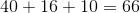The number of students who took the test is the sum of the students who finished in the six ranges: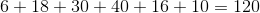The question is now to find out what percent 66 is of 120, which can be calculated as follows: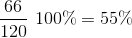### Example Question #7 : Bar GraphsRefer to the above graph. Clarissa, a sixth grader at Polk, scored a 673 on the math portion of the SCAT. Which of the following could have been her rank among the students?